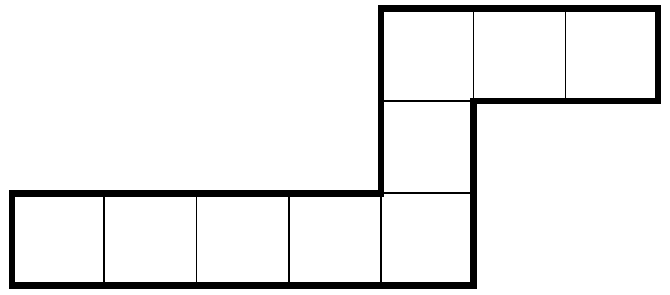###### back to index | new

Let $\mathbb{S}$ be the set of integers between $1$ and $2^{40}$ that contain two $1$s when written in base $2$. What is the probability that a random integer from $\mathbb{S}$ is divisible by $9$?

(Thue's theorem) Let $p$ be a prime. Show that for any integer $a$ such that $p\not\mid a$, there exist positive integers $x$, $y$ not exceeding $\lfloor{\sqrt{p}}\rfloor$ satisfying $ax\equiv y\pmod{p}$ or $ax\equiv -y\pmod{p}$.

Find the smallest integer $N$ such that $\varphi(n) \ge 5$ holds for all integer $n \ge N$.

Let $p$ be a prime. Show that there exist infinitely many positive integer $n$ such that $p\mid (2^n-n)$.

Show that there are infinite many composite numbers in the sequence $$1, 31, 331, 3331, 33331, \cdots$$

Let $n$ be a positive integer. Show that there exist $n$ consecutive integers each of which contains a divisor who is a square number greater than $1$.

Let $p$ be a prime number and $\lfloor{x}\rfloor$ denote the largest integer not exceeding real number $x$. Show that $$C_n^p\equiv\left\lfloor{\frac{n}{p}}\right\rfloor\pmod{p}$$

Solve the following relation in integers: $$x^2 + a^2 = (x+1)^2 + b^2 = (x+2)^2 + c^2 = (x+3)^2 + d^2$$

Let integer $N=\left\lfloor{(\sqrt{29}+\sqrt{21})^{2020}}\right\rfloor$ where $\lfloor{x}\rfloor$ denotes the largest integer not exceeding $x$. Find the last two digits of $N$.

Let $n$ be a positive integer and function $S_1(n)$ return the square of the sum of $n$'s digits. Additionally, let $S_{k+1}(n)=S_1\left(S_k(n)\right)$, where $k$ is a positive integer. Find the value of $S_{1991}(2^{1990})$.

Let $m$ and $n$ be two positive integers, find the minimal value of $\mid 12^m - 5^n\mid$.

Find all ordered integer pairs $(x, y)$ such that $x^3 + y^3=2019$.

Find all the integer pairs $(x, y)$ such that $x^3 = 2^y + 15$.

Let $a$, $b$, and $x_0$ all be positive integers. Sequence $\{x_n\}$ is defined as $x_{n+1}=ax_n + b$ where $n \ge 1$. Show that $x_1$, $x_2$, $\cdots$ cannot be all prime.

Determine all positive integer $n$ such that the following equation is solvable in integers: $$x^n + (2+x)^n + (2-x)^n = 0$$

Let $n$ be an integer which is divisible by neither $2$ nor $5$. Show that $n$ must be divisible by a number whose digits are all $1$.

There are $5$ red balls, $4$ green balls and $3$ yellow balls in a bag. One ball is retrieved a time until all the balls are taken out. What is the probability that all the red balls are retrieved before all the green or yellow balls are retrieved?

Let sequence $\{a_n\}$ be $a_n=2^n + 3^n + 6^n - 1$ where $n\ge 1$. Find the sum of all positive integers which are co-prime to all the $a_n$.

Find, with proof, all ordered pairs of positive integers $(a, b)$ with the following property: there exist positive integers $r$, $s$, and $t$ such that for all $n$ for which both sides are defined, $$\binom{\binom{n}{a}}{b}=r\binom{n+s}{t}$$

$\textbf{Eel}$

An eel is a polyomino formed by a path of unit squares that makes two turns in opposite directions. (Note that this means the smallest eel has four cells.) For example, the polyomino shown below is an eel. What is the maximum area of a $1000\times 1000$ grid of unit squares that can be covered by eels without overlap?Let $f(x)=a_0+a_1x+a_2x^2+\cdots +a_nx^n$ be a $n$-degree polynomial and all its coefficients $a_i$ $(0\le i\le n)$ be either $1$ or $-1$. If $f(x)$ has only real roots, what is the maximum value of $n$?

Show that $$\frac{1}{(1-x)^n}=\sum_{k=0}^{\infty}\binom{n-1+k}{n-1}x^k$$

Let $m$ and $n$ be positive integers satisfying $1 < m < n$. Show that $(1+m)^n > (1+n)^m$.

Show that for any positive integer $n$, the value of $\displaystyle\sum_{k=0}^{n}2^{3k}\binom{2n+1}{2k+1}$ is not a multiple of $5$.

Let $n$, $r$, and $m$ all be positive integers, $r\le m$, and $\omega_k=e^{\frac{2k\pi}{m}i}$ be a complex root to the equation $x^m=1$. Show $$\sum_{k=0}^{\lfloor{\frac{n-r}{m}}\rfloor}\binom{n}{r+km}x^{r+km}=\frac{1}{m}\sum_{k=0}^{m-1}\omega^{-r}(1+x\omega_k)^n$$

where function $\lfloor{x}\rfloor$ returns the largest integer not exceeding real number $x$.